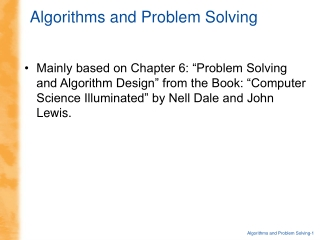DownloadDownload PresentationAlgorithms and Problem Solving

# Algorithms and Problem Solving

Download Presentation## Algorithms and Problem Solving

- - - - - - - - - - - - - - - - - - - - - - - - - - - E N D - - - - - - - - - - - - - - - - - - - - - - - - - - -
##### Presentation Transcript

1. Algorithms and Problem Solving • Mainly based on Chapter 6: “Problem Solving and Algorithm Design” from the Book: “Computer Science Illuminated” by Nell Dale and John Lewis.

2. Objectives • Determine whether a problem is suitable for a computer solution • Describe the computer problem-solving process and relate it to Polya’s How to Solve It list • Distinguish between following an algorithm and developing one • Apply top-down design methodology to develop an algorithm to solve a problem • Apply the object-oriented design methodology to develop a collection of interacting objects to solve a problem

3. Problem Solving • Problem solving is the act of finding a solution to a perplexing, distressing, vexing, or unsettled question

4. Problem Solving • G. Polya wrote How to Solve It: A New Aspect of Mathematical Method • His How to Solve It list is quite general • Written in the context of solving mathematical problems • The list becomes applicable to all types of problems

5. Problem Solving and Algorithms • Ask questions to understand the problem • Look for familiar things • Divide and conquer • Test your solution

6. Ask Questions... • …to understand the problem • What do I know about the problem? • What is the information that I have to process in order the find the solution? • What does the solution look like? • What sort of special cases exist? • How will I recognize that I have found the solution?

7. Look for Familiar Things • You should never reinvent the wheel • In computing, you see certain problems again and again in different guises • A good programmer sees a task, or perhaps part of a task (a subtask), that has been solved before and plugs in the solution

8. Divide and Conquer • Break up a large problem into smaller units that we can handle • Applies the concept of abstraction • The divide-and-conquer approach can be applied over and over again until each subtask is manageable

9. Algorithms • An algorithm is defined as a finite set of steps, each of which may require one or more operations and if carried out on a set of inputs, will produce one or more outputs after a finite amount of time.

10. The Computer Problem-Solving Process

11. Pseudocode • Uses a mixture of English and formatting to make the steps in the solution explicit

12. Following an Algorithm • Preparing a Hollandaise sauce

13. Following an Algorithm (cont.) • Preparing a Hollandaise sauce Page 150

14. Developing an Algorithm • The plan must be suitable in a suitable form • Two methodologies that are currently in use: • Top-down design • Object-oriented design

15. Top-Down Design • Breaking the problem into a set of subproblems called modules • Creating a hierarchical structure of problems and subproblems

16. Top-Down Design • This process continues for as many levels as it takes to expand every task to the smallest details • A step that needs to be expanded is an abstract step Figure 6.5 An example of top-down design

17. A General Example • Planning a large party